# Bellman Ford’s Algorithm

Amit Kumar Ghosh  18 min read
19 Jun 2023
460 Views

## Introduction

Do you know what the Bellman-Ford Algorithm is? If not, don't worry it's actually simpler than it sounds! The Bellman-Ford Algorithm is a powerful tool that helps us find the shortest paths in networks. This algorithm can help you understand the implications of multiple connections and how they determine efficient travel routes or optimal cost networks. In this article, we will explore an overview of the Bellman-Ford Algorithm to get a better understanding of its basic principles and practical applications. After reading this article, you'll gain a clearer grasp of how this algorithm works and why it matters for networking solutions that rely on precise calculations and efficient use of resources.

## What is Bellman Ford Algorithm

Bellman Ford Algorithm is a fundamental algorithm in computer science that helps to solve a range of important problems, from finding the shortest path between two points in a graph to calculating the best way to route traffic on a network. At its core, the algorithm works by building up a set of "distance estimates" for each node in a graph, gradually refining them until every node has the best possible estimate. While the algorithm may seem complex at first glance, its ability to solve a wide variety of problems has made it a crucial tool for many fields, from computer science to civil engineering

## Application of Bellman Ford's Algorithm

1. Routing protocols: The Bellman-Ford algorithm is widely used in computer networks as the basis for distance-vector routing protocols. These protocols are responsible for determining the best path for data packets to travel through a network. Examples of routing protocols that use the Bellman-Ford algorithm include the Routing Information Protocol (RIP) and the Border Gateway Protocol (BGP).
2. Network topology analysis: The Bellman-Ford algorithm can be used to analyze and understand the topology of a network. By running the algorithm on a network graph, it can determine the shortest path and associated costs between different nodes. This information is valuable for network administrators to optimize network performance and identify potential bottlenecks.
3. Internet Service Providers (ISPs): ISPs use the Bellman-Ford algorithm for network traffic engineering and to ensure efficient routing of data across their networks. By applying the algorithm to the network topology, ISPs can determine the best path for data packets to reach their destination, considering factors such as link capacities, congestion, and network policies.
4. Distance vector protocols in wireless sensor networks: Wireless sensor networks often have limited resources, such as battery power and computing capabilities. The Bellman-Ford algorithm can be implemented in these networks to compute the shortest path while considering energy constraints and other network-specific requirements.
5. Virtual Private Networks (VPNs): The Bellman-Ford algorithm is used in VPNs to determine the optimal path for network traffic between different nodes in the VPN infrastructure. It helps establish secure and efficient communication between geographically distributed networks.
6. Resource allocation in cloud computing: The Bellman-Ford algorithm can be used to allocate resources in cloud computing environments. By modeling the cloud infrastructure as a graph, the algorithm can find the shortest path and associated costs between different resources, such as servers, storage, and network components. This information can assist in optimizing resource allocation and load balancing.

## Bellman Ford Algorithm Example

Here's an example of the Bellman-Ford algorithm in action:

Let's consider a directed weighted graph with the following edges and their respective weights:

• Edge 1: A -> B, weight = 2
• Edge 2: A -> C, weight = 4
• Edge 3: B -> C, weight = 1
• Edge 4: B -> D, weight = 7
• Edge 5: C -> D, weight = 3
• Edge 6: D -> A, weight = 5

We want to find the shortest paths from a source vertex (let's say A) to all other vertices in the graph.

Step 1: Initialization

We set the distance to the source vertex as 0, and all other distances as infinity.

• Distance to A: 0
• Distance to B: Infinity
• Distance to C: Infinity
• Distance to D: Infinity

Step 2: Relaxation

We iterate over all the edges |V| - 1 time, where |V| is the number of vertices in the graph, and relax the edges. In each iteration, we update the distances if we find a shorter path.

Iteration 1:

• Distance to A: 0
• Distance to B: 2
• Distance to C: 4
• Distance to D: Infinity

Iteration 2:

• Distance to A: 0
• Distance to B: 2
• Distance to C: 3
• Distance to D: 6

Iteration 3:

• Distance to A: 0
• Distance to B: 2
• Distance to C: 3
• Distance to D: 6

Iteration 4:

• Distance to A: 0
• Distance to B: 2
• Distance to C: 3
• Distance to D: 6

Iteration 5:

• Distance to A: 0
• Distance to B: 2
• Distance to C: 3
• Distance to D: 6

Step 3: Negative cycle detection

After |V| - 1 iterations, we check for any negative cycles in the graph. In this example, there are no negative cycles.

Step 4: Result

The final distances from the source vertex A to all other vertices are as follows:

• Distance to A: 0
• Distance to B: 2
• Distance to C: 3
• Distance to D: 6

So, the shortest path from A to B is 2, from A to C is 3, and from A to D is 6.

## How Bellman Ford's Algorithm Works?

Bellman-Ford's algorithm is a shortest path algorithm used to find the shortest paths from a source vertex to all other vertices in a weighted directed graph. It can handle graphs with negative edge weights, unlike Dijkstra's algorithm, which requires non-negative weights. The algorithm works as follows:

1. Initialize the distance values for all vertices in the graph as infinity, except for the source vertex, which is set to 0. Also, initialize the predecessor of all vertices as undefined.
2. Relaxation step: Repeat the following process |V|-1 times, where |V| is the number of vertices in the graph.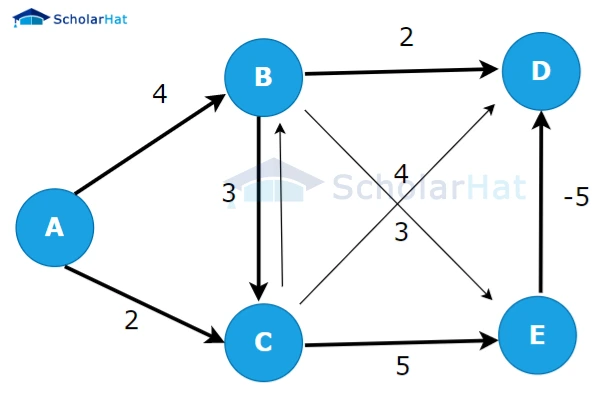3.  For each edge (u, v) in the graph, where u is the source vertex and v is the destination vertex:
• If the distance from the source vertex to u plus the weight of the edge (u, v) is less than the current distance value of v, update the distance of v with this new value.
• Also, update the predecessor of v as u.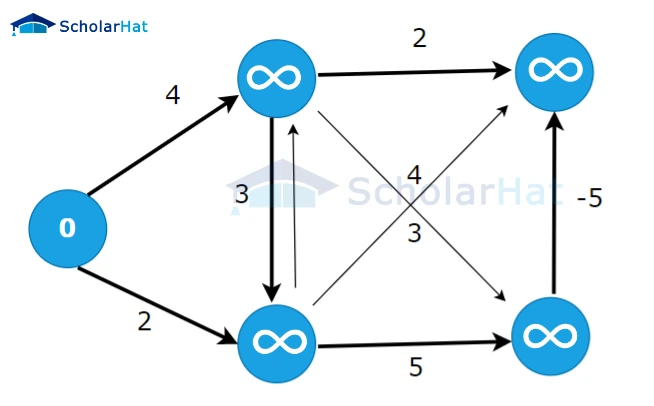4. Check for negative-weight cycles: Repeat the following process for each edge (u, v) in the graph.
• If the distance from the source vertex to u plus the weight of the edge (u, v) is less than the current distance value of v, then there is a negative-weight cycle in the graph.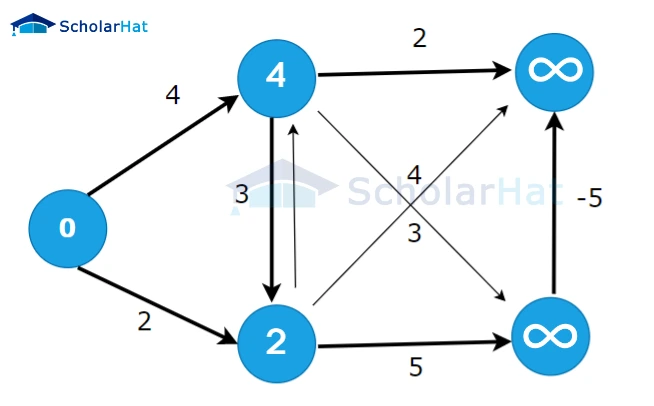5. After completing the relaxation step |V|-1 times, the algorithm will have calculated the shortest distances from the source vertex to all other vertices in the graph (if no negative-weight cycles exist). If there is a negative-weight cycle, step 3 will detect it.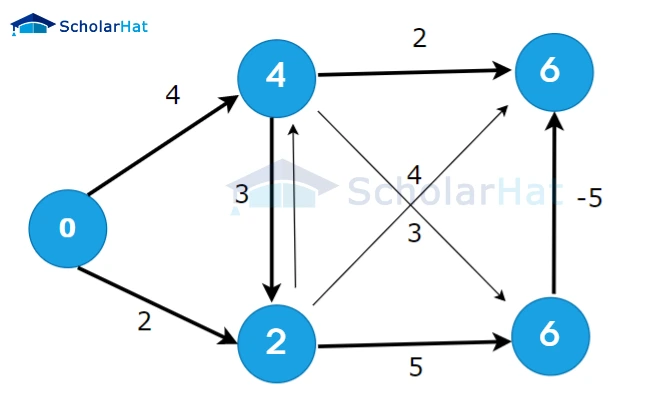6. If required, the shortest paths can be reconstructed using the predecessor information. Starting from the destination vertex, follow the predecessor pointers until reaching the source vertex to obtain the shortest path.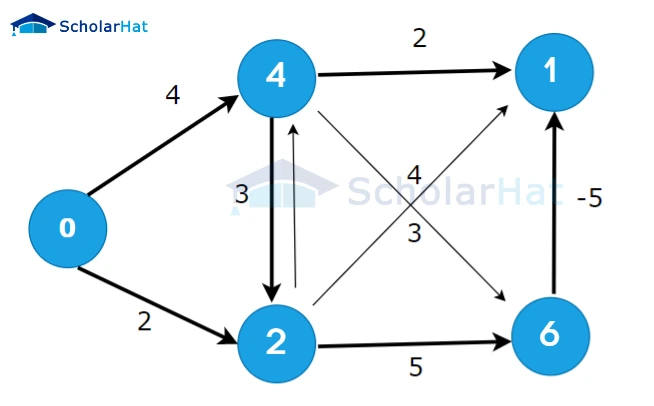7. The algorithm guarantees the correct shortest path distances if there are no negative-weight cycles reachable from the source vertex. However, if there is a negative-weight cycle that can be reached from the source vertex, the algorithm will detect it in step 3. In such cases, the algorithm cannot provide meaningful shortest path distances, as the negative-weight cycle allows for infinite negative weight reductions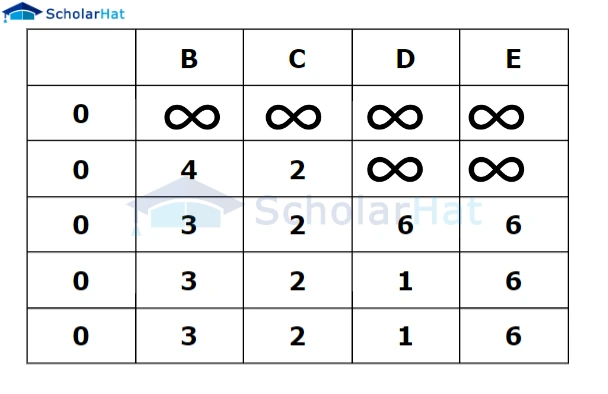## Bellman Ford Algorithm Pseudocode

Bellman Ford algorithm, which is used to find the shortest paths from a single source vertex to all other vertices in a weighted directed graph. The algorithm can handle negative edge weights but detects negative cycles.

``````function BellmanFord(Graph, source):
// Step 1: Initialization
for each vertex v in Graph:
distance[v] := infinity
predecessor[v] := null
distance[source] := 0

// Step 2: Relaxation
for i from 1 to |V|-1:
for each edge (u, v) in Graph:
if distance[u] + weight(u, v) < distance[v]:
distance[v] := distance[u] + weight(u, v)
predecessor[v] := u

// Step 3: Check for negative cycles
for each edge (u, v) in Graph:
if distance[u] + weight(u, v) < distance[v]:
return "Graph contains a negative cycle"

// Step 4: Output the shortest paths
return distance[], predecessor[]``````

In this pseudocode:

• The graph represents the input graph.
• The source is the source vertex from which to find the shortest paths.
• distance[v] represents the shortest distance from the source to vertex v.
• predecessor[v] stores the predecessor of vertex v in the shortest path.

The algorithm consists of four main steps:

1. Initialization: Set the distance of all vertices to infinity, except for the source vertex, which is set to 0. Set the predecessor of all vertices to null.
2. Relaxation: Perform a relaxation step for each edge in the graph |V|-1 times. The relaxation step updates the distance to each vertex v if there exists a shorter path through vertex u.
3. Check for negative cycles: Perform one more iteration to check for negative cycles. If a shorter path can still be found, then there exists a negative cycle in the graph.
4. Output the shortest paths: After completing the algorithm, the shortest distances and predecessors are returned.

Note that |V| represents the number of vertices in the graph, and weight(u, v) returns the weight/cost of the edge (u, v).

## Implementation Code in Java, Python, and C++ Python Java C++ `````` import sys # Class for representing edges of the graph class Edge:     def __init__(self, u, v, w):         self.u = u # Start vertex of the edge         self.v = v # End vertex of the edge         self.w = w # Weight of the edge (u, v) # Class for representing the graph class Graph:     def __init__(self, V, E):         self.V = V # Total number of vertices in the graph         self.E = E # Total number of edges in the graph         self.edges = [] # List of edges # Bellman-Ford algorithm implementation def bellmanFord(graph, u):     V = graph.V     E = graph.E     dist = [sys.maxsize] * V     # Step 1: Initialize the distance array     dist[u] = 0     # Step 2: Relax edges |V| - 1 times     for _ in range(V - 1):         for j in range(E):             edge = graph.edges[j]             if dist[edge.u] != sys.maxsize and dist[edge.u] + edge.w < dist[edge.v]:                 dist[edge.v] = dist[edge.u] + edge.w     # Step 3: Check for negative weight cycles     for j in range(E):         edge = graph.edges[j]         if dist[edge.u] != sys.maxsize and dist[edge.u] + edge.w < dist[edge.v]:             print("Graph contains negative weight cycle")             return     # No negative weight cycle found     # Print the distance array     printArr(dist, V) def printArr(arr, size):     for i in range(size):         print(arr[i], end=" ")     print() # Create a graph V = 5 # Total vertices E = 8 # Total edges # Create an instance of the graph graph = Graph(V, E) # Adding the edges of the graph """     edge(u, v)     where u = start vertex of the edge (u,v)             v = end vertex of the edge (u,v)     w is the weight of the edge (u,v) """ # Edge 0 --> 1 graph.edges.append(Edge(0, 1, 5)) # Edge 0 --> 2 graph.edges.append(Edge(0, 2, 4)) # Edge 1 --> 3 graph.edges.append(Edge(1, 3, 3)) # Edge 2 --> 1 graph.edges.append(Edge(2, 1, 6)) # Edge 3 --> 2 graph.edges.append(Edge(3, 2, 2)) bellmanFord(graph, 0) # 0 is the source vertex``````

#### Output

``````
0 5 4 8 10
This output represents the shortest distances from the source vertex (vertex 0) to all other vertices (vertices 1 to 4) in the graph. The distances are as follows:
Distance from vertex 0 to vertex 0: 0
Distance from vertex 0 to vertex 1: 5
Distance from vertex 0 to vertex 2: 4
Distance from vertex 0 to vertex 3: 8
Distance from vertex 0 to vertex 4: 10
The code does not detect any negative weight cycles in the graph, so it does not print the "Graph contains negative weight cycle" message.``````

## The Complexity of Bellman Ford Algorithm

The Bellman-Ford algorithm has a time complexity of O(V * E), where V represents the number of vertices and E represents the number of edges in the graph.

In the algorithm, the main idea is to relax each edge repeatedly for a total of V-1 times, where V is the number of vertices in the graph. During each relaxation step, the algorithm updates the distance to each vertex by considering all outgoing edges from the current vertex. This process of bellman ford algorithm time complexity is repeated V-1 times to ensure that the shortest paths are correctly computed.

Since, in the worst case, each edge is relaxed V-1 times, the total number of relaxation steps is V-1 times the number of edges, i.e., O(V * E). Therefore, the time complexity of the Bellman-Ford algorithm is O(V * E).

It is worth noting that the algorithm can also detect negative cycles by performing an additional iteration of relaxation. If there is a negative cycle reachable from the source vertex, the algorithm will indicate the presence of the cycle. However, if there are no negative cycles, the algorithm terminates after the V-1 iterations, making the time complexity O(V * E).

##### Summary

The Bellman-Ford Algorithm is a fundamental algorithm used to find the shortest paths in a graph, considering the weights or costs associated with each edge. It is commonly used in computer networks and routing protocols. Overall, the Bellman-Ford Algorithm is a versatile and widely used algorithm for solving shortest path problems in various domains, enabling efficient routing and resource allocation in networks.

Similar Articles